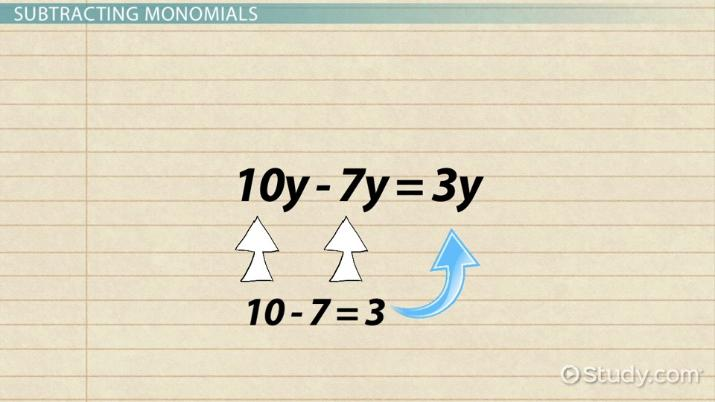# How to Add & Subtract Monomials

Lesson Transcript
Instructor: Yuanxin (Amy) Yang Alcocer

Amy has a master's degree in secondary education and has been teaching math for over 9 years. Amy has worked with students at all levels from those with special needs to those that are gifted.

Monomials are single terms with a coefficient multiplied with variables. Learn more about monomials and how only like terms can be added or subtracted. Then, learn about adding and subtracting monomials. Updated: 11/09/2021

## Monomials

In this lesson, we will talk about working with monomials. Monomials are single terms consisting of a coefficient multiplied with variables. Your coefficient is the number that is multiplied by a variable, and your variable is a letter representing an unknown value.

For example, in the monomial 4x, the 4 is the coefficient and the x is the variable. This particular monomial only has one variable, but it is also possible to have more than one variable, such as in 3xy, where 3 is the coefficient and x and y are the variables.

It is also possible for your variables to have exponents. 8x^2, for example, is a monomial where 8 is the coefficient and the x^2 is the variable x with an exponent of 2. The exponent tells you how many you have of the variable. So x^2 tells you that you have 2 x's. If your monomial only has variables, such as in x, then your coefficient is automatically a 1.An error occurred trying to load this video.

Try refreshing the page, or contact customer support.

Coming up next: How to Multiply & Divide Monomials

### You're on a roll. Keep up the good work!

Replay
Your next lesson will play in 10 seconds
• 0:02 Monomials
• 1:09 Only Like Terms
• 1:43 Adding Monomials
• 3:30 Subtracting Monomials
• 4:44 Lesson Summary
Save Save

Want to watch this again later?

Timeline
Autoplay
Autoplay
Speed Speed

## Only Like Terms

Now that you know what monomials are, the next most important thing to know is that you can only add or subtract like terms. Like terms are monomials that share the same variables. For example, 4x and 7x are like terms because the variable x is the same in both. The variables have to be exactly the same. 4x and 4x^2 are not like terms because their variables are not exactly the same. The coefficients do not have to be the same.

Now, let's see about adding monomials together. Let's say you are given the problem 4x + 9x. The first thing you need to do is to check to see if your monomials are like terms. You ask yourself, are the variables exactly the same?

Yes, they are. So that means you can go ahead and add them together. To add them together, you leave the variables as they are and you add up the coefficients. So you can add 4 + 9. What does this equal? It equals 4 + 9 = 13. So our answer is 13x. We have the variables exactly the same.

If you are given a problem where you don't have like terms, you will leave the terms that are not alike alone. For example, if your problem involved adding several monomials together as in 2xy + 5xy + 6y, you again first look to see if you have like terms. You see that the 2xy and the 5xy are like terms so that means you can add those two together.

You remember that you leave the variables alone and add the coefficients. You add 2 + 5 = 7; that means 2xy + 5xy = 7xy. But you still have the 6y. What do you do with this one? Because this is not a like term, you can't do anything with it. So, you leave it as it is and your complete answer is 2xy + 5xy + 6y = 7xy + 6y. You have added the like terms and everything else is left alone and included in the answer.

To unlock this lesson you must be a Study.com Member.

### Register to view this lesson

Are you a student or a teacher?

Back

### Resources created by teachers for teachers

Over 30,000 video lessons & teaching resources‐all in one place.Video lessonsQuizzes & WorksheetsClassroom IntegrationLesson Plans

I would definitely recommend Study.com to my colleagues. It’s like a teacher waved a magic wand and did the work for me. I feel like it’s a lifeline.

Jennifer B.
TeacherCreate an account to start this course today
Used by over 30 million students worldwide